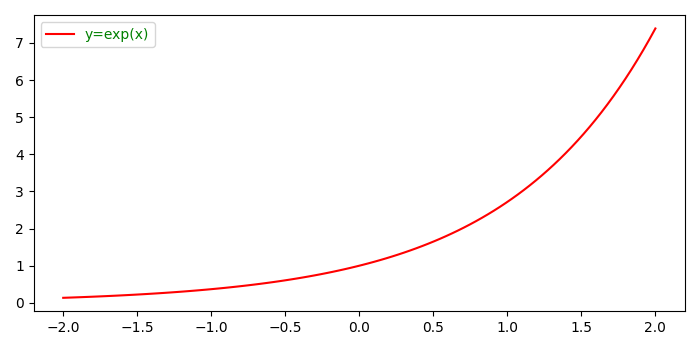# How to change the text color of font in the legend using Matplotlib?

To change the text color of font in the legend in matplotlib, we can take the following steps−

• Create x and y data points using numpy.
• Plot x and y using plot() method, where color of line is red and label is "y=exp(x)".
• To place the legend, use legend() method with location of the legend and store the returned value to set the color of the text.
• To set the color of the text, use set_color() method with green color.
• To display the figure, use show() method.

## Example

import numpy as np
from matplotlib import pyplot as plt
plt.rcParams["figure.figsize"] = [7.00, 3.50]
plt.rcParams["figure.autolayout"] = True
x = np.linspace(-2, 2, 100)
y = np.exp(x)
plt.plot(x, y, label="y=exp(x)", c='red')
leg = plt.legend(loc='upper left')
for text in leg.get_texts():
text.set_color("green")
plt.show()

## Output